Probability: Featured Book!
 Probability: For the Enthusiastic Beginner By David J. Morin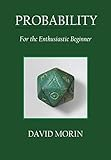This book is written for high school and college students learning about probability for the first time. It will appeal to the reader who has a healthy level of enthusiasm for understanding how and why the various results of probability come about. All of the standard introductory topics in probability are covered: combinatorics, the rules of probability, Bayes’ theorem, expectation value, variance, probability density, common distributions, the law of large numbers, the central limit theorem, correlation, and regression. List Price: \$19.95New: \$18.93Used: \$14.77 Order your copy today!

More Excellent Books on Probability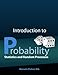Introduction to Probability, Statistics, and Random Processes Hossein Pishro-Nik This book introduces students to probability, statistics, and stochastic processes. It can be used by both students and practitioners in engineering, various sciences, finance, and other related fields. It provides a clear and intuitive approach to these topics while maintaining mathematical accuracy. The book contains a large number of solved exercises. > Buy Now List Price: \$38.50New: \$31.66Used: \$15.65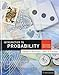Introduction to Probability, 2nd Edition Dimitri P. Bertsekas, John N. Tsitsiklis An intuitive, yet precise introduction to probability theory, stochastic processes, and probabilistic models used in science, engineering, economics, and related fields. The 2nd edition is a substantial revision of the 1st edition, involving a reorganization of old material and the addition of new material. The length of the book has increased by about 25 percent. The main new feature of the 2nd edition is thorough introduction to Bayesian and classical statistics. The book is the currently used textbook for "Probabilistic Systems Analysis," an introductory probability course at the MIT. > Buy Now List Price: \$91.00New: \$86.45Used: \$60.80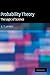Probability Theory: The Logic of Science E. T. Jaynes A Bayesian approach to science. This introduction to probability was written by a scientist for scientists. The author shows how Bayesian theory can be applied to inference from incomplete information. The book is a pleasure to read, especially the author's entertaining arguments against conventional theory. That is not to say that it is an easy read; on the contrary, the math is high-level and the problems are challenging. To follow the author's presentation, the reader will need to be familiar with applied mathematics at an advanced undergraduate level. > Buy Now List Price: \$116.00New: \$70.58Used: \$64.17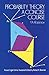Probability Theory: A Concise Course (Dover Books on Mathematics) Y.A. Rozanov This book, a concise introduction to modern probability theory and certain of its ramifications, deals with a subject indispensable to natural scientists and mathematicians alike. Readers, with some knowledge of mathematics, will find an excellent treatment of the elements of probability together with numerous applications. Each of the eight chapters and four appendixes include relevant problems (150 of them), many with hints and answers. > Buy Now List Price: \$11.95New: \$6.82Used: \$1.40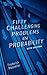Fifty Challenging Problems in Probability with Solutions (Dover Books on Mathematics) Frederick Mosteller Fifty challenging probability problems. Remarkable selection of puzzlers, graded in difficulty, that illustrate both elementary and advanced aspects of probability. Selected for originality, general interest, or because they demonstrate valuable techniques, the problems are ideal as a supplement to courses in probability or statistics, or as stimulating recreation for the mathematically minded. Each problem is illustrated with a detailed solution. If you have a soft spot for problems in probability, this book is an inexpensive must. > Buy Now List Price: \$9.95New: \$5.00Used: \$3.49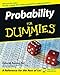Probability For Dummies Deborah J. Rumsey Practical tips for solving probability problems. Increase your chances of acing that probability exam or winning at the casino! Whether you are hitting the books or hitting the tables, working out probabilities can be a challenge. This book helps even the odds. The book covers set theory, counting, permutations and combinations, random variables, conditional probability, joint distributions, conditional expectations, gambling, and actuarial applications and probability modeling. And it is packed with real-life examples and straightforward, friendly explanations. > Buy Now List Price: \$19.99New: \$9.78Used: \$2.13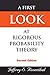First Look At Rigorous Probability Theory, A (2Nd Edition) Jeffrey S Rosenthal This textbook is an introduction to probability theory using measure theory. It is designed for graduate students in a variety of fields (mathematics, statistics, economics, management, finance, computer science, and engineering) who require a working knowledge of probability theory that is mathematically precise, but without excessive technicalities. The text provides complete proofs of all the essential introductory results. Nevertheless, the treatment is focused and accessible, with the measure theory and mathematical details presented in terms of intuitive probabilistic concepts, rather than as separate, imposing subjects. > Buy Now List Price: \$33.00New: \$30.69Used: \$21.23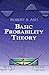Basic Probability Theory (Dover Books on Mathematics) Robert B. Ash Geared toward advanced undergraduates and graduate students, this introductory text surveys random variables, conditional probability and expectation, characteristic functions, infinite sequences of random variables, Markov chains, and an introduction to statistics. Complete solutions to some of the problems appear at the end of the book; specifically, 43 pages of solutions. > Buy Now List Price: \$22.95New: \$10.00Used: \$3.36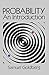Probability: An Introduction Samuel Goldberg Excellent basic text covers set theory, probability theory for finite sample spaces, binomial theorem, probability distributions, means, standard deviations, probability function of binomial distribution, and other key concepts and methods essential to a thorough understanding of probability. Designed for use by math or statistics departments offering a first course in probability. 360 illustrative problems with answers for half. Only high school algebra needed. > Buy Now List Price: \$18.95New: \$11.04Used: \$0.10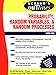Schaum's Outline of Probability, Random Variables, and Random Processes Hwei Hsu Pages and pages of solved probability problems. Designed for students in various disciplines of engineering, science, mathematics, management, and business, this effective study tool includes hundreds of problems with step-by-step solutions and another 150 problems with hints or delayed answers. The solved problems illustrate and strongly reinforce vital theory and techniques. Topics taught include functions of random variables, expectation and limit theorems, estimation theory, decision theory, and queuing theory. > Buy Now List Price: \$19.95New: \$7.24Used: \$2.00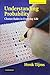Understanding Probability: Chance Rules in Everyday Life Henk Tijms A fresh approach to probability. A pleasant surprise; a book that makes probability theory easy to understand. The author uses colorful examples (gambling, lotteries, etc.) and historical references (Newton, Einstein, etc.) to illustrate basic principles and stimulate reader interest. This book very clearly explains why probability works and how to apply it. > Buy Now List Price: \$48.00New: \$47.63Used: \$7.24